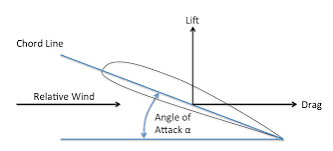# How do I get angle of attack?

Angle of attack is a core component of the lift equation and coefficients in terms of aerodynamics.

How would I use something like Vector3:Dot() to get the difference in angle between the direct the wingpart is going and the direction its facing?The angle of attack is the angle between the relative wind and the chord line of the airfoil. The relative wind is the relative motion of the body of your aircraft against oncoming airflow. If you know the direction your part is going and the direction your part is pointing toward, the angle of attack can be represented as:

``````local aoa = math.acos(math.clamp(partMotionDirection:Dot(partFacingDirection), -1, 1))
``````

If you know the part’s velocity along its roll/longitudinal axis and its yaw/perpendicular axis, another representation I saw was:

``````local aoa = math.atan2(v_yaw, v_roll)
``````

I don’t know much regarding this subject, so if I am wrong, someone correct me. (Also, I assumed still air, but if your project has varying winds, your direction of motion would be relative to those winds.)

2 Likes

literally been trying for years to figure out what the hell a lift coefficient is and when I see angle of attack I’m like, holy crap, that’s the key! Thank you so much!

I have a question, basically, how do I only get the AoA in pitch, instead of all directions? Like, just the Y axis, and not any other ones?

It’s better to use the second formula. In the first one, I also forgot to account for a negative angle of attack. You will need to convert the motion direction to object space. It will ignore the motion in the local x direction.

``````local relPartMotionDirection = part.CFrame:VectorToObjectSpace(partMotionDirection)
local v_yaw = relPartMotionDirection.Y
local v_roll = -relPartMotionDirection.Z -- front facing is negative Z direction
local aoa = -math.atan2(v_yaw, v_roll) -- relative vertical velocity will be negative when climbing, and vice versa when descending
``````